## MOS Transistor Definitions

• n-type MOS: Majority carriers are electrons.
• p-type MOS: Majority carriers are holes.

• Positive/negative voltage applied to the gate (with respect to substrate) enhances the number of electrons/holes in the channel and increases conductivity between source and drain.

• V t defines the voltage at which a MOS transistor begins to conduct. For voltages less than V t (threshold voltage), the channel is cut off.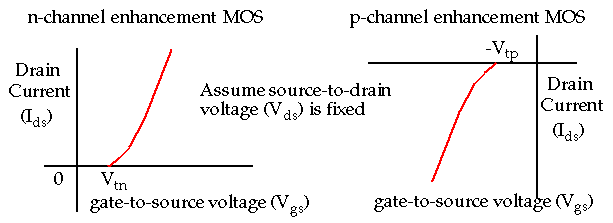## MOS Transistor Definitions

• In normal operation, a positive voltage applied between source and drain (V ds ).
• No current flows between source and drain (I ds = 0) with V gs = 0 because of back to back pn junctions.

• For n-MOS, with V gs > V tn , electric field attracts electrons creating channel.
• Channel is p-type silicon which is inverted to n-type by the electrons attracted by the electric field.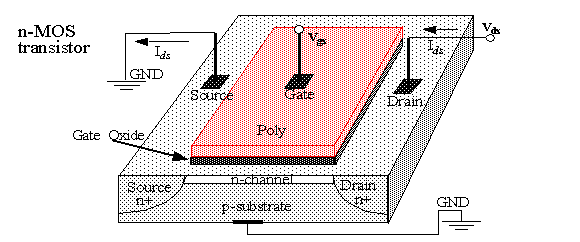## n-MOS Enhancement Transistor Physics

• Three modes based on the magnitude of V gs : accumulation, depletion and inversion.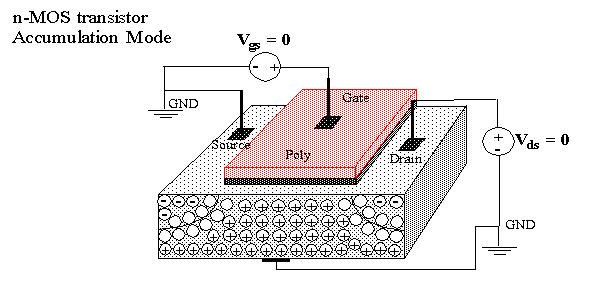## n-MOS Enhancement Transistor Physics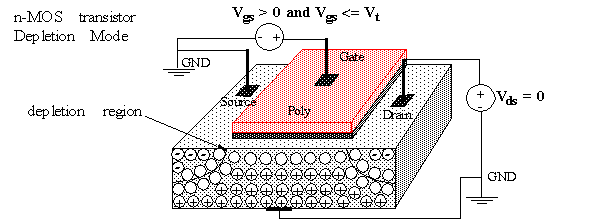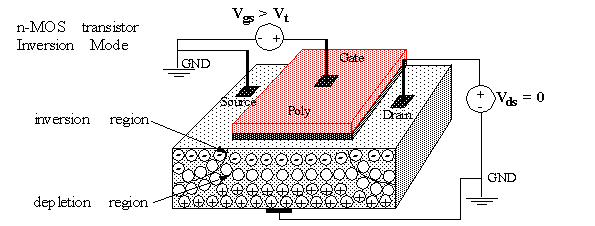## n-MOS Enhancement Transistor

• With V ds non-zero, the channel becomes smaller closer to the drain.

• When V ds <= V gs - V t (e.g. V ds = 3V, V gs = 5V and V t = 1V), the channel reaches the drain (since V gd > V t ).

• This is termed linear , resistive or nonsaturated region. I ds is a function of both V gs and V ds .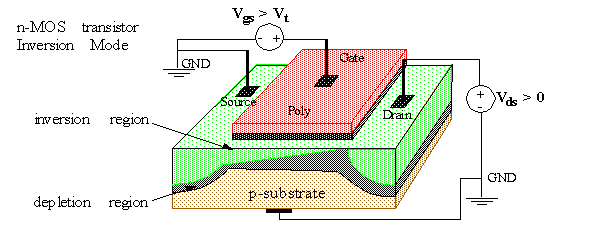## n-MOS Enhancement Transistor

• When V ds > V gs - V t (e.g. V ds = 5V, V gs = 5V and V t = 1V), the channel is pinched off close to the drain (since V gd < V t ).

• This is termed saturated region. I ds is a function of V gs , almost independent of V ds .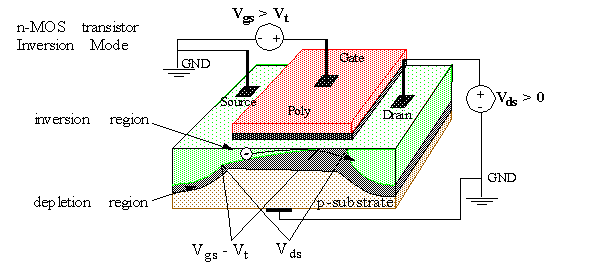## MOS Enhancement Transistor

• MOS transistors can be modeled as a voltage controlled switch. I ds is an important parameter that determines the behavior, e.g., the speed of the switch.

• What are the parameters that effect the magnitude of I ds ? (Assume V gs and V ds are fixed, e.g. 5V).
• The distance between source and drain (channel length).
• The channel width.
• The threshold voltage.
• The thickness of the gate oxide layer.
• The dielectric constant of the gate insulator.
• The carrier (electron or hole) mobility.

• Summary of normal conduction characteristics:
• Cut-off : accumulation, I ds is essentially zero.
• Nonsaturated : weak inversion, I ds dependent on both V gs and V ds .
• Saturated : strong inversion, I ds is ideally independent of V ds .

## Threshold Voltage

• V t is also an important parameter. What effects its value?

• Most are related to the material properties. In other words, V t is largely determined at the time of fabrication, rather than by circuit conditions, like I ds .

• For example, material parameters that effect V t include:
• The gate conductor material (poly vs. metal).
• The gate insulation material (SiO 2 ).
• The thickness of the gate material.
• The channel doping concentration.

• However, V t is also dependent on
• V sb (the voltage between source and substrate), which is normally 0 in digital devices.
• Temperature: changes by -2mV/degree C for low substrate doping levels.

## Threshold Voltage

• The expression for threshold voltage is given as: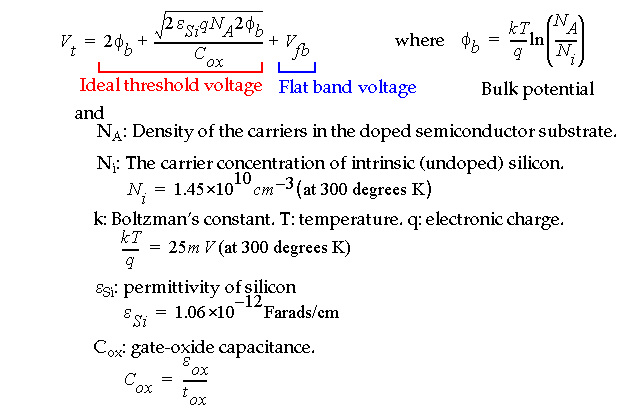## Threshold Voltage

• Threshold voltage (cont.):• Typical values of V t for n and p-channel transistors are +/- 700mV.

## Threshold Voltage

• From equations, threshold voltage may be varied by changing:
• The doping concentration (N A ).
• The oxide capacitance (C ox ).
• Surface state charge (Q fc ).

• As you can see, it is often necessary to adjust V t .
• Two methods are common:
• Change Q fc by introducing a small doped region at the oxide/substrate interface via ion implantation.

• Change C ox by using a different insulating material for the gate.
• A layer of Si 3 N 4 (silicon nitride) with a relative permittivity of 7.5 is combined with a layer of silicon dioxide (relative permittivity of 3.9).
• This results in a relative permittivity of about 6.

• For the same thickness dielectric layer, C ox is larger using the combined material, which lowers V t .

## Body Effect

• In digital circuits, the substrate is usually held at zero.
• The sources of n-channel devices, for example, are also held at zero, except in cases of series connections, e.g.,• The source-to-substrate (V sb ) may increase at this connections, e.g. V sbN1 = 0 but V sbN2 /= 0.
• V sb adds to the channel-substrate potential: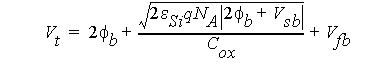## Basic DC Equations

• Ideal first order equation for cut-off region: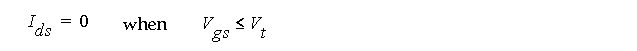• Ideal first order equation for linear region: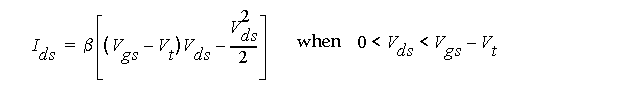• Ideal first order equation for saturation region: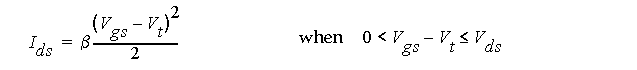• with the following definitions:## Basic DC Equations

• Process dependent factors: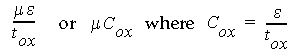.

• Geometry dependent factors: W and L.

• Voltage-current characteristics of the n- and p-transistors.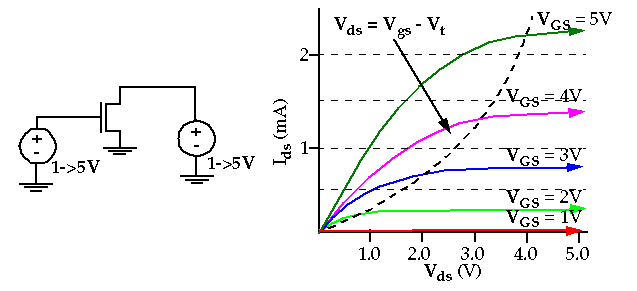## Beta calculation

• Transistor beta calculation example:
• Typical values for an n-transistor in 1 micron technology: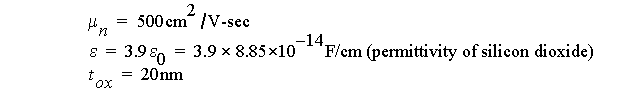• Compute beta:• How does this beta compare with p-devices:• n-transistor gains are approximately 2.8 times larger than p-transistors.

## Inverter voltage transistor characteristics

• Inverter DC characteristics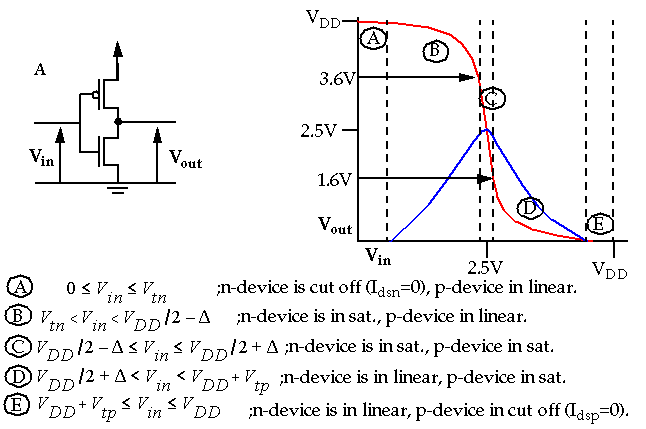## Beta Ratios

• Region C is the most important region. A small change in the input voltage, V in , results in a LARGE change in the output voltage, V out .

• This behavior describes an amplifier, the input is amplified at the output. The amplification is termed transistor gain, which is given by beta.

• Both the n and p-channel transistors have a beta. Varying their ratio will change the characteristics of the output curve.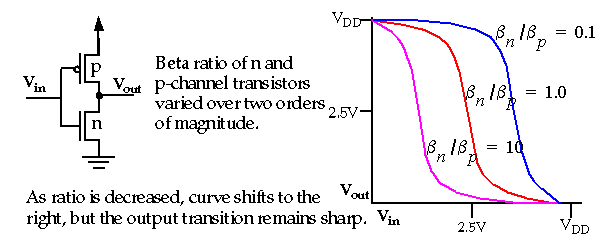## Beta Ratios

• Therefore, the
•• does NOT affect switching performance.

• What factor would argue for a ratio of 1 for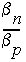?
• Load capacitance !

• The time required to charge or discharge a capacitive load is equal when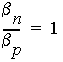.

• Since beta is dependent W and L, we can adjust the ratio by changing the sizes of the transistor channel widths, by making p-channel transistors wider than n-channel transistors.

## Noise Margins

• A parameter that determines the maximum noise voltage on the input of a gate that allows the output to remain stable.

• Two parameters, Low noise margin (NM L ) and High noise margin (NM H ).

• NM L = difference in magnitude between the max LOW output voltage of the driving gate and max LOW input voltage recognized by the driven gate.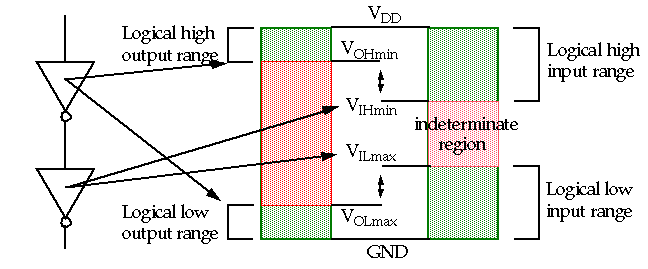## Noise Margins

• Ideal characteristic: V IH = V IL = (V OH +V OL )/2.

• This implies that the transfer characteristic should switch abruptly (high gain in the transition region).

• V IL found by determining unity gain point from V OH .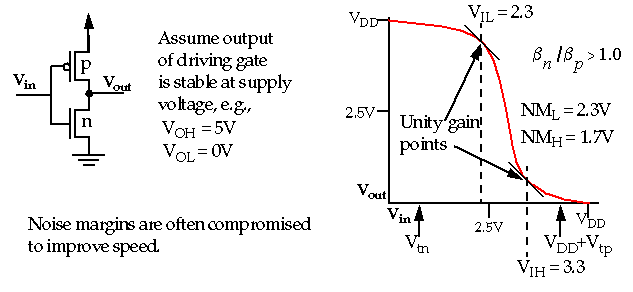## Pseudo-nMOS Inverter• Therefore, the shape of the transfer characteristic and the V OL of the inverter is affected by the ratio.
• In general, the low noise margin is considerably worse than the high noise margin for Pseudo-nMOS.

• Pseudo-nMOS was popular for high-speed circuits, static ROMs and PLAs.

## Pseudo-nMOS

• Example: Calculation of noise margins: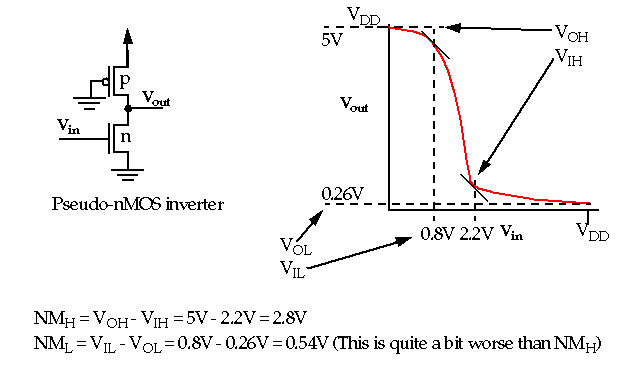• The transfer curve for the pseudo-nMOS inverter can be used to calculate the noise margins of identical pseudo-nMOS inverters.